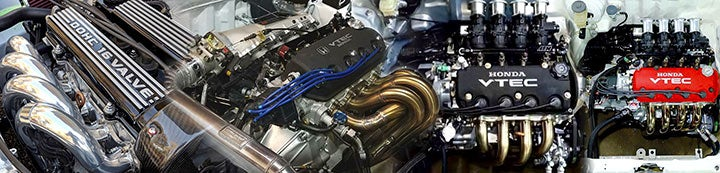1 - 5 of 5 Posts

icarusdown

·
Registered
Joined
·
1,402 Posts
Discussion Starter
you get nothing!

99EJ6T

·
Registered
Joined
·
14,661 Posts
Moudy said:
How to plot points on a compressor map:

Calculate airflow for the X axis: measured in lb/min, airflow = (HP target) x (air/fuel ratio) x (BSFC/60) where BSFC is lbs fuel / (hp x hr) If you don’t have access to BSFC data, you can plug in an estimated value between .50-.60.

Calculate volumetric efficiency = (actual CFM / theoretical CFM) x 100 where theoretical CFM = (RPM x CID) / 3456 and actual CFM = 90%, a good average number if you don’t have access to measuring this. Multiply by .0610237 to convert cubic centimeter displacement into cubic inches.

Calculate manifold absolute pressure: map = [(airflow) x (639.6) x (460 + intake temperature)] / (VE) x (RPM/2) x (CID) Upwards of 100 degrees F is a good estimate for intercooled intake temps if you don’t have access to measuring this. Plan on adding about 1psi to take into account pressure drops related to the intake and/or intercooler.

Calculate pressure ratio for the Y axis = (14.7 + map) / 14.7 If you’re at sea level, 14.7psi will work. As elevation varies, this figure will need to be adjusted.

Note: Don’t worry about the constants like 3456, 60 or 639.6, they’re simply there to tidy things up and convert units.

VE = volumetric efficiency
BSFC = brake specific fuel consumption
HP = projected flywheel horsepower
CFM = cubic feet per minute
CID = cubic inch displacement

Other useful calculations:

Trim = (minor/major)squared x 100

Trim describes the relationship the minor diameter and major diameter of a compressor or turbine wheel shares with each another. The inducer, or minor end when referring to compressor wheels, is where the air enters. Air exits through the exducer portion.
full post: here

•icarusdown and superdewey58

superdewey58

·
i shoot stuff
Joined
·
2,422 Posts
nice

•ddd4114, 99EJ6T and mindless

99EJ6T

·
Registered
Joined
·
14,661 Posts
thanks for the link.
there was some nice, clear information there that helped me understand a little more about compressor maps.
yep, no problem.

Neez

·
Registered
Joined
·
57 Posts
good info. thanks

1 - 5 of 5 Posts